# LineFlow 开源：比 PyTorch 简洁数倍，适用任何框架的 NLP 数据集处理程序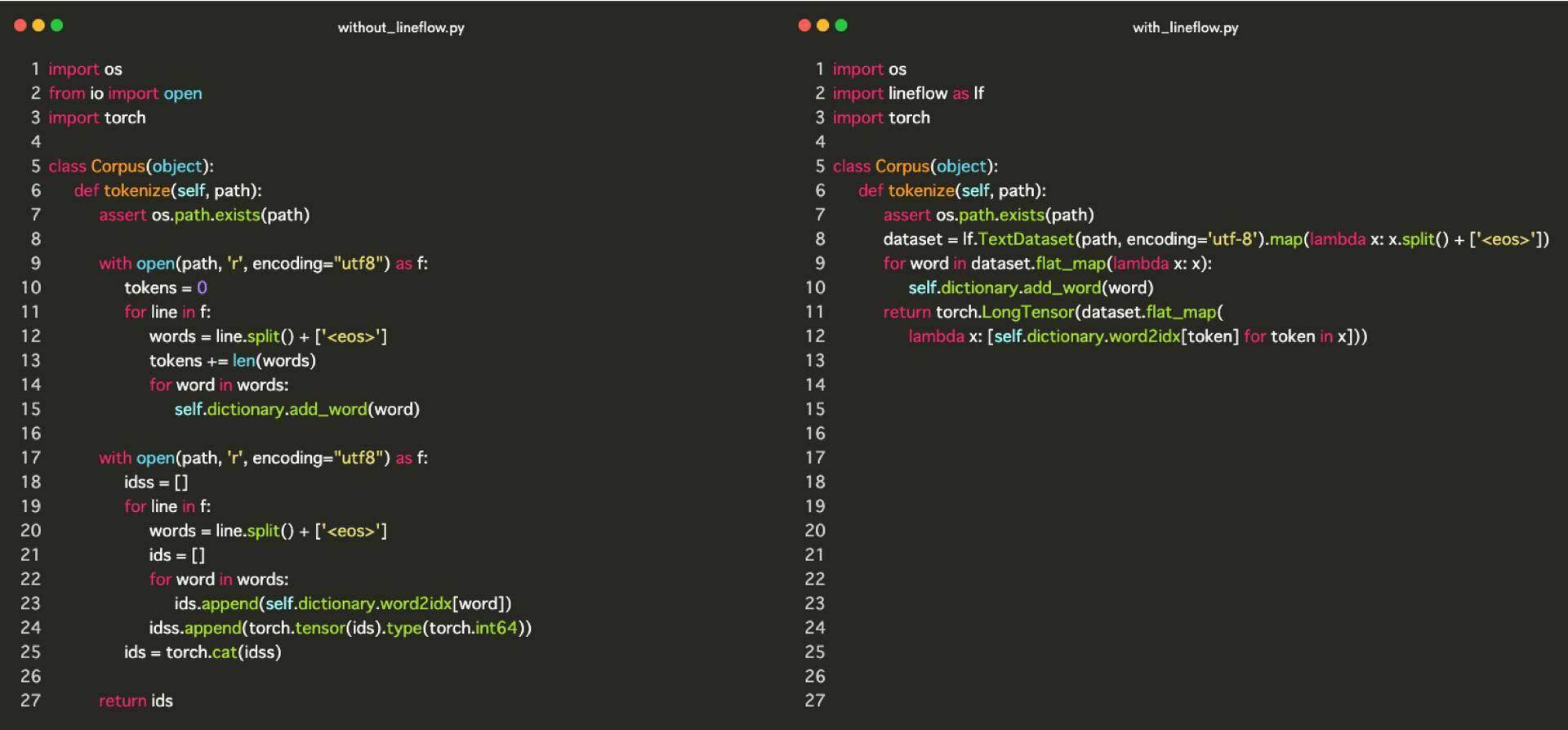## 加载文本数据

dataset = lf.TextDataset(path, encoding='utf-8').map(...)

lf.TextDataset 要求的数据格式是每行对应一个数据。如果文本数据满足此条件，则可以加载任何类型的文本数据。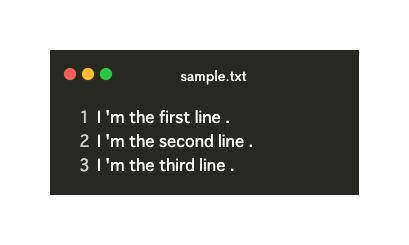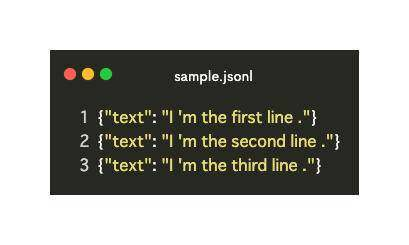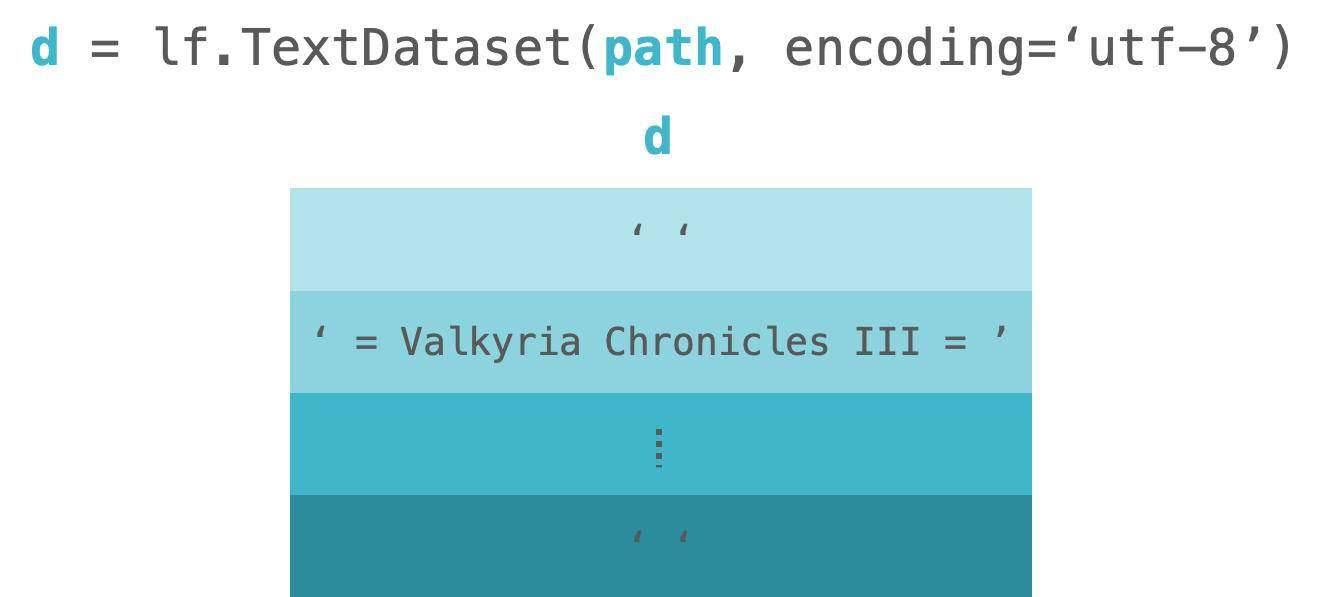LineFlow 已经提供了一些公开可用的数据集。所以你可以马上使用它。要查看提供的数据集，请访问此链接

## 2. 标记化

dataset = lf.TextDataset(...).map(lambda x: x.split() + ['<eos>'])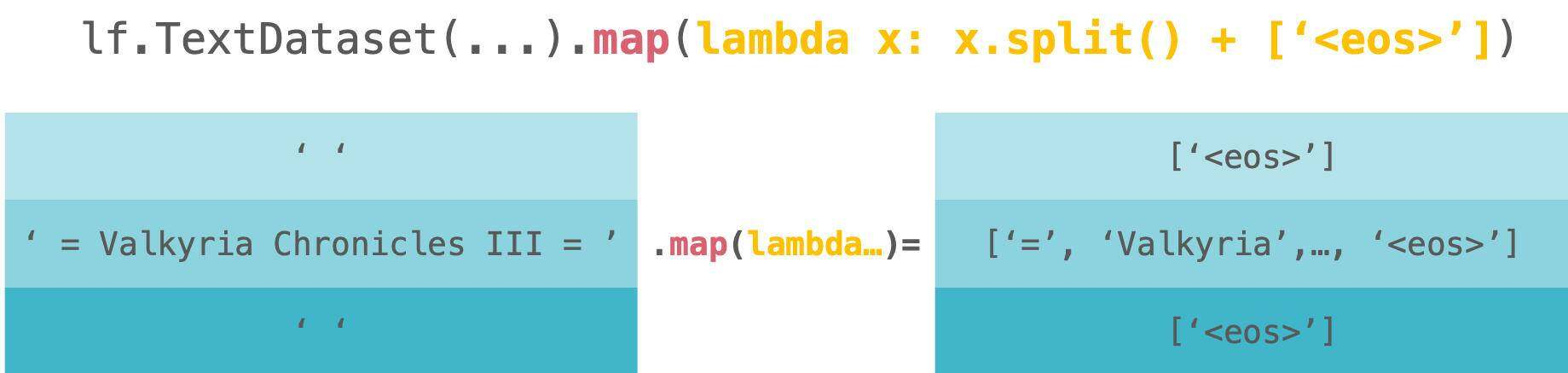lambda x: x.split() + ['<eos>']

>>> from blingfire import text_to_words>>> d = lf.TextDataset('/path/to/your/text')>>> d.map(text_to_words).map(str.split)

>>> d = lf.TextDataset('/path/to/text')>>> d.map(tokenize).map(lambda x: (x, len(x)))

## 3. 索引

for word in dataset.flat_map(lambda x: x):    self.dictionary.add_word(word)return torch.LongTensor(dataset.flat_map(...))

for word in dataset.flat_map(lambda x: x):    self.dictionary.add_word(word)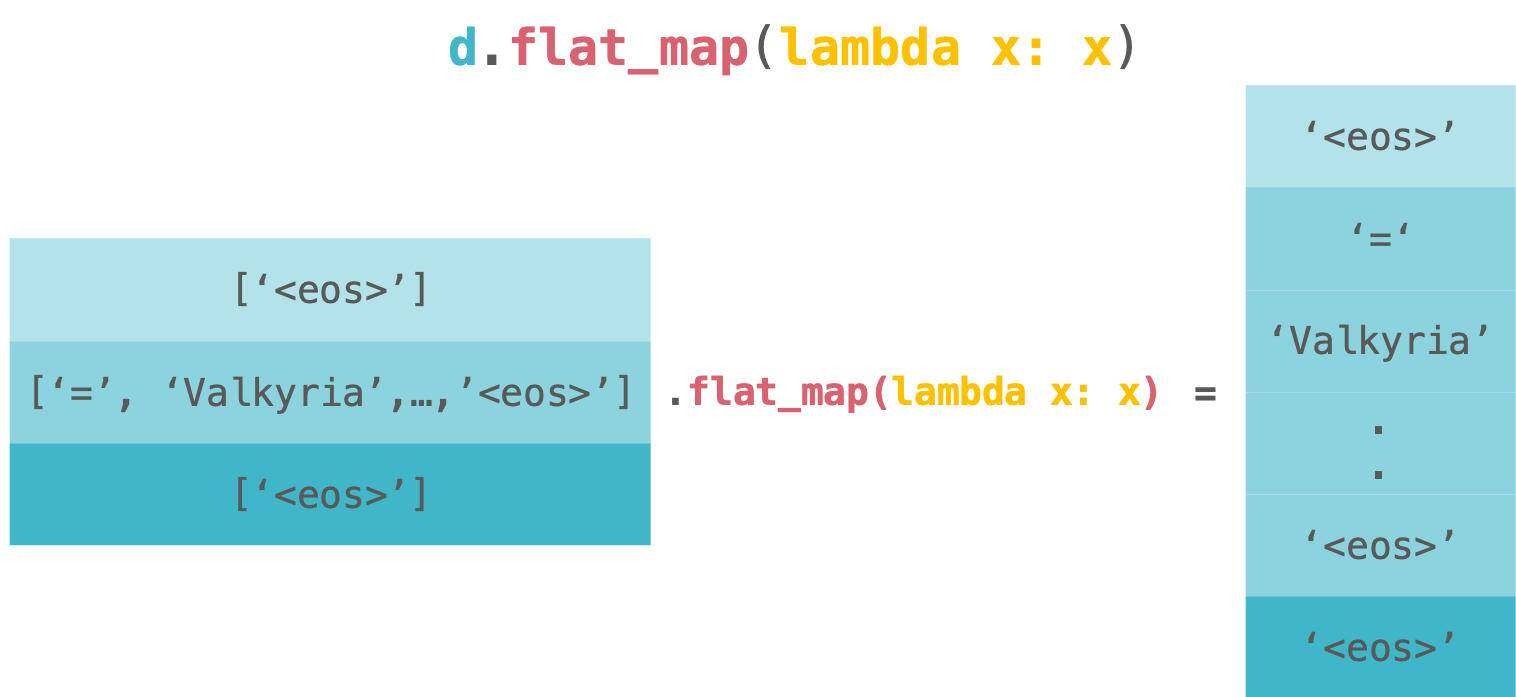flat_map 有点令人困惑，但它等同于下面的代码。

>>> from itertools import chain>>> chain.from_iterable(map(lambda x: x, dataset))>>>>>> dataset.flat_map(lambda x: x) # same as above

self.dictionary.add_word(word)

dataset.flat_map(    [lambda x: self.dictionary.word2idx[token] for token in x)])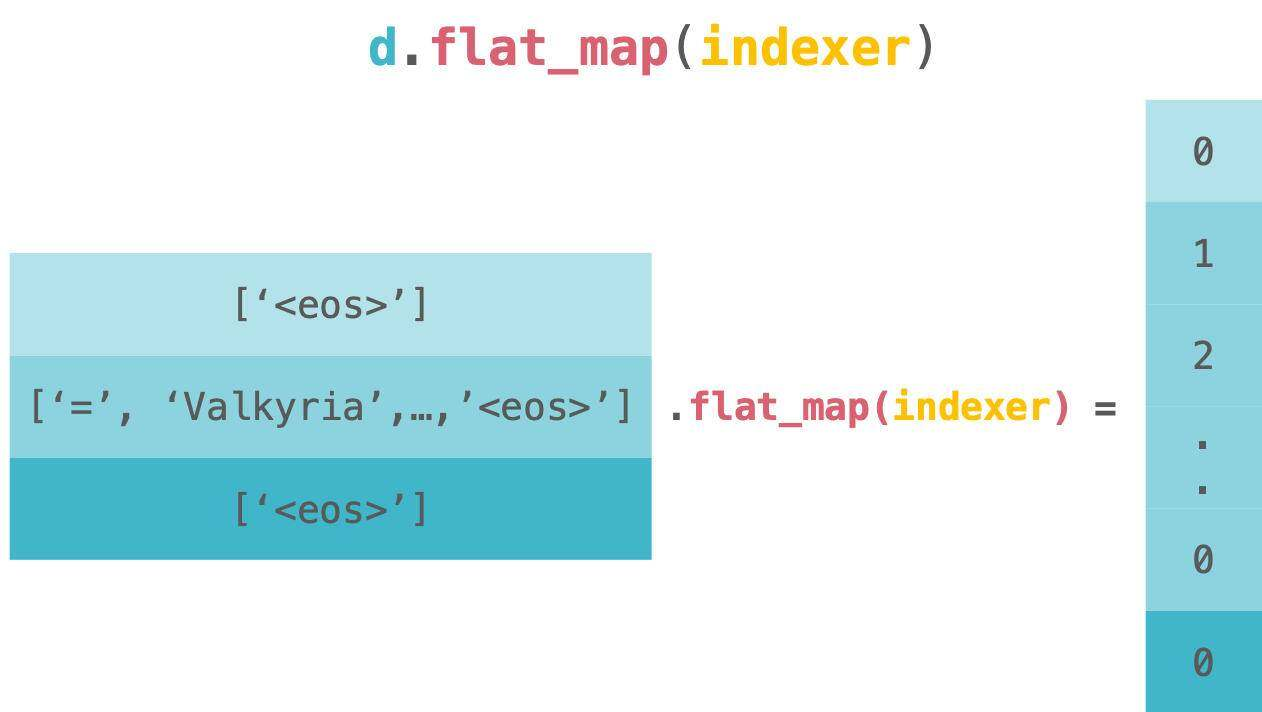>>> from itertools import chain>>> chain.from_iterable(map(indexer, dataset))>>>>>> dataset.flat_map(indexer) # same as above

return torch.LongTensor(dataset.flat_map(...))

import osimport torchimport lineflow as lfclass Dictionary(object):    def __init__(self):        self.word2idx = {}        self.idx2word = []    def add_word(self, word):        if word not in self.word2idx:            self.idx2word.append(word)            self.word2idx[word] = len(self.idx2word) - 1        return self.word2idx[word]    def __len__(self):        return len(self.idx2word)class Corpus(object):    def __init__(self, path):        self.dictionary = Dictionary()        self.train = self.tokenize(os.path.join(path, 'train.txt'))        self.valid = self.tokenize(os.path.join(path, 'valid.txt'))        self.test = self.tokenize(os.path.join(path, 'test.txt'))    def tokenize(self, path):        assert os.path.exists(path)        dataset = lf.TextDataset(path, encoding='utf-8').map(lambda x: x.split() + ['<eos>'])        for word in dataset.flat_map(lambda x: x):            self.dictionary.add_word(word)        return torch.LongTensor(dataset.flat_map(            lambda x: [self.dictionary.word2idx[token] for token in x]))`

Yasufumi TANIGUCHI，软件工程师，对自然语言处理有着浓厚的兴趣。本文最初发表于 Medium 博客，经原作者 Yasufumi TANIGUCHI 授权，InfoQ 中文站翻译并分享。

https://towardsdatascience.com/lineflow-introduction-1caf7851125e